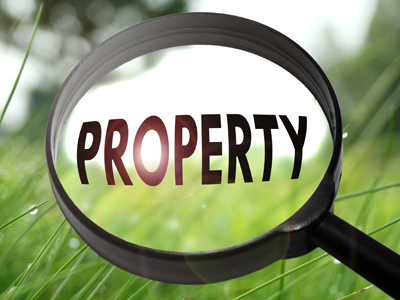This quiz investigates the properties of numbers.

# Properties of Numbers 3 (Medium)

Welcome to the third of our Medium level Eleven Plus maths quizzes on Properties of Numbers. If you have played the previous ones then you should know some of these, like multiples, primes and squares. This quiz gives you more opportunity to practise and cement your knowledge of different terms.

Here are some things to remember:

• Odd – whole number not divisible by 2
• Even – whole number divisible by 2
• Prime – divisibly only by 1 and by itself
• Square – the result of a number multiplied by itself
• Multiple – can be divided by named number (e.g. 8 is a multiple of 4)

Okay then, let’s get to it! Take your time and think about the answers before you choose which one you think is correct. If you have been practising with our other quizzes, this one should be a doddle!

1.
Which of the following statements is true?
7 is a prime number
4 is a multiple of 8
30 is a square number
An odd number plus an even number equals an even number
30 is not a square number: 5 x 5 = 25, 6 x 6 = 36
4 is not a multiple of 8, though 8 is a multiple of 4: 4 x 2 = 8
An odd number plus an even number equals an odd number.
7 is a prime number because it is only divisible by 1 and by 7: 7 x 1 = 7, 1 x 7 = 7
2.
If a number is divisible by 8, what must the final digit of the number be?
8
6 or 8
2, 4, 6 or 8
0, 2, 4, 6 or 8
Numbers in the 8 times table all end with an even number or with 0
3.
Which of the following is a square number?
66
88
100
111
A square number is formed by multiplying another number with itself : 10 x 10 = 100
4.
What happens to an even number if you multiply it by 6?
It becomes an odd number
It remains an even number
It could be either odd or even
There are no rules
When we multiply two even numbers (6 is an even number) the result is always an even number – try it yourself to see
5.
If a number is divisible by 9, what must the final digit of the number be?
9
6, 7, 8 or 9
1, 3, 5, 7 or 9
0, 1, 2, 3, 4, 5, 6, 7, 8 or 9
As 9 is an odd number, its multiples can end with any number. Go through your 9 times table and you’ll see this is true
6.
Which of these numbers is a multiple of 24?
4
6
12
48
If a number is a multiple of another number, then it is divisible by the other number exactly a certain number of times.
4, 6 and 12 go into 24 exactly:
4 x 6 = 24, 6 x 4 = 24 and 12 x 2 = 24 - but that means 24 is a multiple of those numbers. Only 48 is a multiple of 24 because 24 x 2 = 48
7.
What happens to an odd number if you multiply it by 3?
It remains an odd number
It becomes an even number
It could be either odd or even
There are no rules
When we multiply two odd numbers (3 is an odd number) the result is always an odd number – try it yourself to see
8.
Which of the following statements is wrong?
If a number is divisible by 6, it is an even number
If a number is divisible by 14, it is divisible by 7
If a number is divisible by 6, it is divisible by 12
If a number is a multiple of 12, it is divisible by both 3 and 4
3 x 6 = 18 BUT 18 is NOT divisible by 12. If you want to show that a statement is not always true, find one example that shows it is wrong - like we did here
9.
What do you always get when you add a negative whole number and a negative whole number?
A positive number
A negative number
Either a positive or negative number
Either a positive number or negative number or zero
Negative plus negative equals negative:
For example: -2 + -2 = -4
10.
Which words do not describe the numbers 8, 16, 24 and 32?
Even numbers
Prime numbers
Whole numbers
Multiples of eight
They are all whole even numbers and multiples of 8. Prime numbers are not divisible by any number except one and themselves, so the only even prime number is 2
Author:  Frank Evans